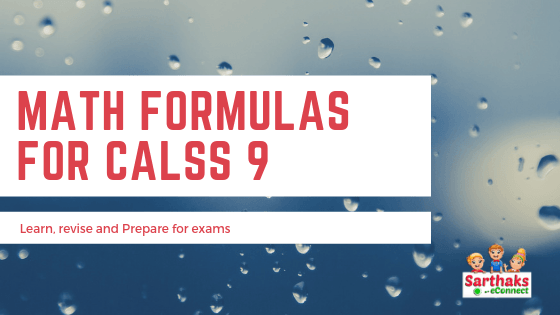All the important math formula for class 9 from each chapter of mathematics is listed here.
The link for formulas related to that chapter is provided on the right side.

# Math formula for class 9:

In this article, we have provided all the essential formulas. It is designed for your convenience so that you can reach it directly and sort out any of your problems easily. Math formula for class 9 includes all the necessary chapters which you need most for your board exams and also daily revision. You don’t have have to move further. You may also note that the important topics from each chapter are also mentioned so that your eye catches it much easier.

## Notes:

Math formula for class 9 also includes little notes with each topic so that if you forgot the terms related to specific formulas then you don’t have to search this in books. To save your precious time and score better for exams we are working. Read each essential math formula.